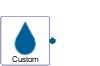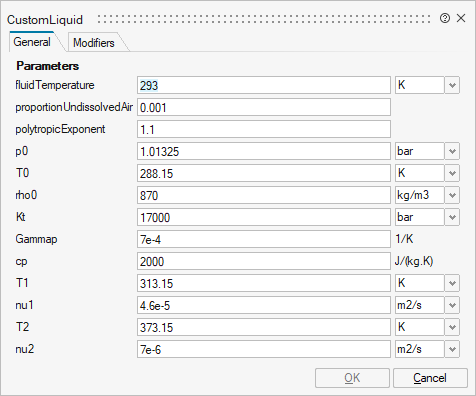# CustomLiquid

Basic graphical layout of input/output block## Library

HydraulicsByFluidon/Media

## Description

Model of a custom hydraulic liquid. The model provides the other components (e.g. pipes) with user-defined temperature- and pressure-dependent fluid properties. In order for the fluid properties to reach the other components, the liquid model must be connected to the hydraulic circuit.

The underlying model is based on the following assumptions:

• In the vicinity of the Reference pressure p0 and Reference temperature T0, the density changes linearly with pressure p and temperature T. Hence, the Isothermal bulk modulus and the Thermal expansion coefficient are constants.
• The change of kinematic viscosity with respect to temperature follows the law proposed by Ubbelohde and Walther (DIN 51563).
• The kinematic viscosity is not affected by the pressure level.
• The specific thermal capacity at constant pressure is a constant.

The density is calculated by the following equation:The isentropic bulk modulus is given by:In order to use the component, the following parameters have to be provided by the user:

• Reference pressure p0 and temperature T0 to calculate the density
• Density rho0 at Reference pressure and temperature
• Isothermal bulk modulus Kt and Thermal expansion coefficient Gammap
• Specific heat capacity cp at constant pressure
• Kinematic viscosities nu1 and nu2 at ambient pressure for two different temperatures T1 and T2
Because the Isothermal bulk modulus is assumed to be constant, the stiffening of the hydraulic fluid with respect to pressure is not taken into account by the employed model. Therefore, the custom liquid should only be used for pressures and temperatures in the vicinity of the reference values p0 and T0.

If the effects of undissolved air are to be considered during the simulation, the user can provide the fraction of undissolved air at ambient pressure through the parameter Proportion of undissolved air. The stiffness of the gas bubbles is calculated based on the parameter Polytropic exponent which can assume values between 1.0 (isothermal change of state, slow compression/expansion) and 1.4 (isentropic change of state, fast compression/expansion).

## ParametersNameLabelDescriptionData TypeValid Values

mo_fluidTemperature

fluidTemperature

Scalar

mo_proportionUndissolvedAir

proportionUndissolvedAir

Proportion of undissolved air

Scalar

mo_polytropicExponent

polytropicExponent

Polytropic exponent

Scalar

mo_p0

p0

Reference pressure for density calculation

Scalar

mo_T0

T0

Reference temperature for density calculation

Scalar

mo_rho0

rho0

Density of fluid at reference temperature and pressure

Scalar

mo_Kt

Kt

Isothermal bulk modulus

Scalar

mo_Gammap

Gammap

Thermal expansion coefficient

Scalar

mo_cp

cp

Specific heat capacity at constant pressure

Scalar

mo_T1

T1

First reference temperature for viscosity calculation

Scalar

mo_nu1

nu1

Kinematic viscosity at T1 and ambient pressure

Scalar

mo_T2

T2

Second reference temperature for viscosity calculation

Scalar

mo_nu2

nu2

Kinematic viscosity at T2 and ambient pressure

Scalar

## Ports

NameTypeDescriptionIO TypeNumber

fluidPort

implicit

Hydraulic port

input

1